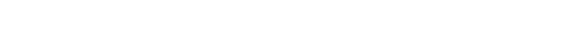# Metric Definitions

## Average Order Value (AOV)

Average Order Value is the average revenue generate from each order that resulted from ads. It's calculated by dividing your total sales by your total orders.Average Order Value data can be found by enabling the AOV column.

## Conversion Rate (CVR)

Conversion rate is the percent of clicks that result in an order. It's calculated by dividing total orders by total clicks.Conversion rate data can be found by enabling the CVR column.

## Ideal CPC

The Ideal CPC use your conversion rate and average order value to calculate your ideal CPC based on your target ACOS. It's calculated by multiplying your target ACOS, average order value, and conversion rate together.Ideal CPC data can be found by enabling the Ideal CPC column.## Cameron Scot

Merch Jar Cofounder

;